# Re-introduction to gghighlight: Highlight ggplot2 with Predicates

## June 16, 2018 by Hiroaki Yutani

Half a year ago, I’ve introduced gghighlight package. I didn’t expect so much R people get interested in my package. Thanks for your attention!

But, please forget about that gghighlight; gghighlight has become far more powerful and simple! So, let me re-introduce about gghighlight.

(Note that this version of gghighlight is not yet on CRAN at the time of this writing. Please install by devtools::install_github("yutannihilation/gghighlight") for the time being)

## Motivation

### dplyr has filter()

What do you do when you explore a data that is too large to print?

library(dplyr, warn.conflicts = FALSE)

big_data %>%
group_by(some_key) %>%
summarise(some_agg = some_func(some_column))
# Opps, the result is too large!


dplyr’s filter() is the Swiss army knife for this, which enables us to narrow down the data. One nice thing of this function is that it can be inserted to any steps in the chain of %>%, so we don’t need to rewrite the entire code.

big_data %>%
# OK, let's filter the data
filter(some_column > some_value) %>%
group_by(some_key) %>%
summarise(some_agg = some_func(some_column))


### ggplot2?

OK, good. But, what about ggplot2?

For a data that has too many series, it is almost impossible to identify a series by its colour as their differences are so subtle.

library(tidyverse)

set.seed(2)
d <- map_dfr(
letters,
~ data.frame(
idx = 1:400,
value = cumsum(runif(400, -1, 1)),
type = .,
flag = sample(c(TRUE, FALSE), size = 400, replace = TRUE),
stringsAsFactors = FALSE
)
)

ggplot(d) +
geom_line(aes(idx, value, colour = type))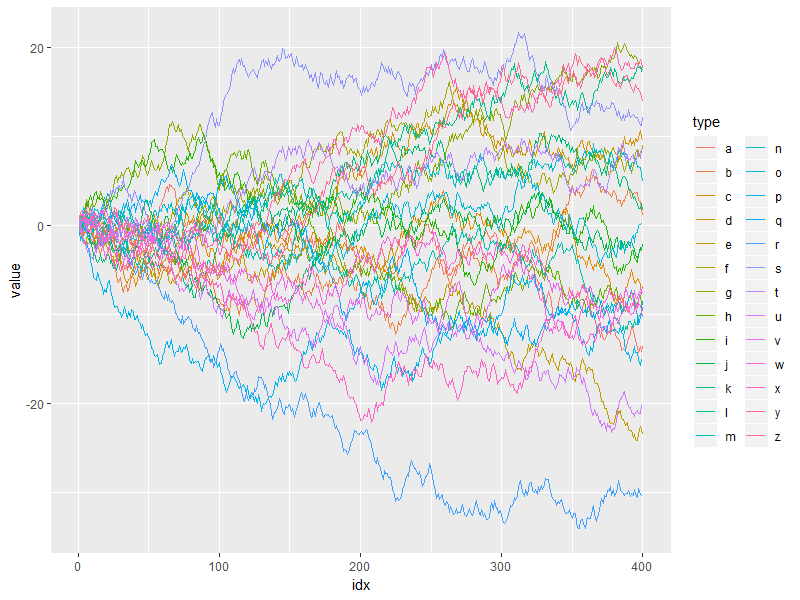Of course, I can use dplyr’s filter() here as well.

library(dplyr, warn.conflicts = FALSE)

d_filtered <- d %>%
group_by(type) %>%
filter(max(value) > 20) %>%
ungroup()

ggplot(d_filtered) +
geom_line(aes(idx, value, colour = type))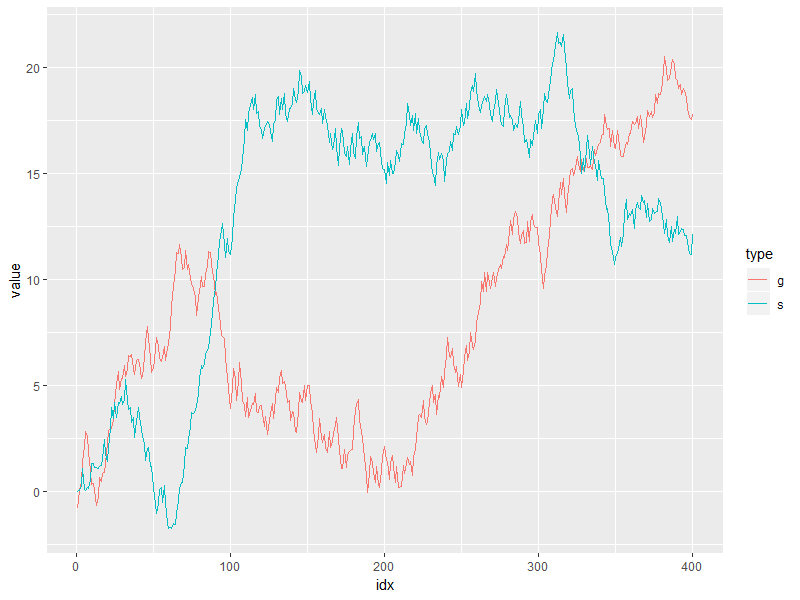But, it seems not so handy. For example, what if I want to change the threshold in predicate (max(value) > 20) and highlight other series as well? It’s a bit tiresome to type all the code above again every time I replace 20 with some other value…

So, I want filter() for ggplot2. This is my initial impulse to create gghighlight.

### Highlighting is better than filtering

In my understanding, one of the main purposes of visualization is to get the overview of a data. In this sense, it may not be good to simply filter out the unmatched data because the plot loose its context then. It’s better to keep the unimportant data as grayed-out lines. Here comes the need for highlighting, like this:

ggplot(d_filtered) +
geom_line(aes(idx, value, group = type), data = d, colour = alpha("grey", 0.7)) +
geom_line(aes(idx, value, colour = type))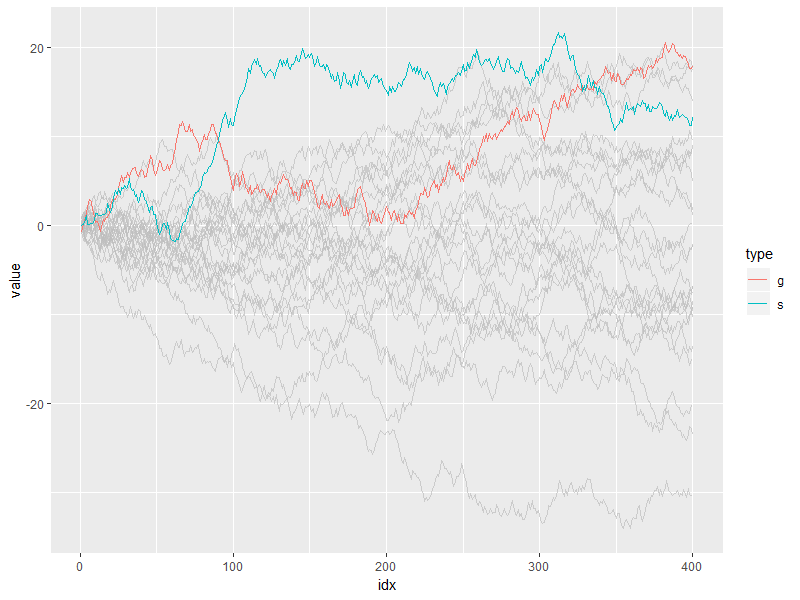This looks nicer! So, now, my motivation has changed a bit; I want a function that highlights the important parts of a data, instead of filtering out the unimportant parts.

(If you are interested in the more details behind the idea of highlighting, you may find this post useful: Anatomy of gghighlight.)

## gghighlight()

Here is my answer, gghighlight():

library(gghighlight)

ggplot(d) +
geom_line(aes(idx, value, colour = type)) +
gghighlight(max(value) > 20)
#> label_key: type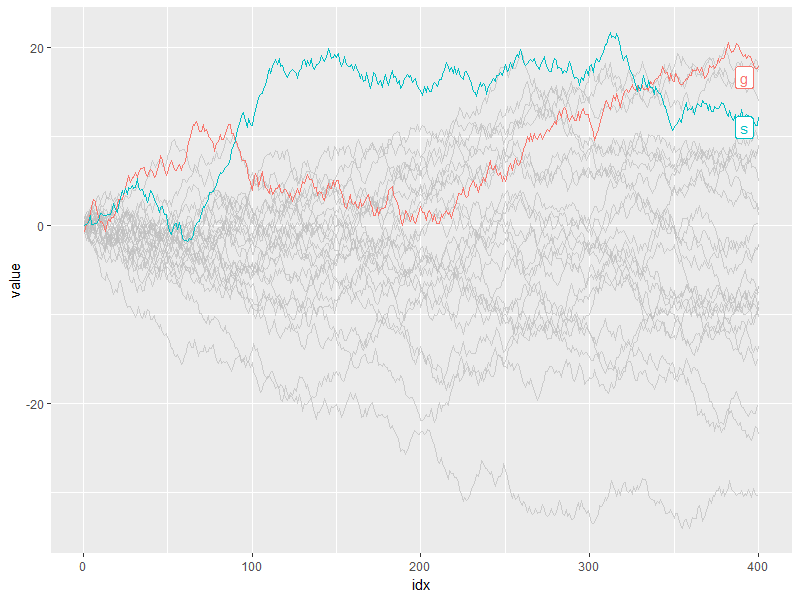Like filtering data with filter(), you can highlight the data by just adding gghighlight().

Just like filter(), you can specify as many predicates as you like. For example, the following code highlights the data that satisfies both max(value) > 15 and mean(flag) > 0.55.

ggplot(d) +
geom_line(aes(idx, value, colour = type)) +
gghighlight(max(value) > 15, mean(flag) > 0.55)
#> label_key: type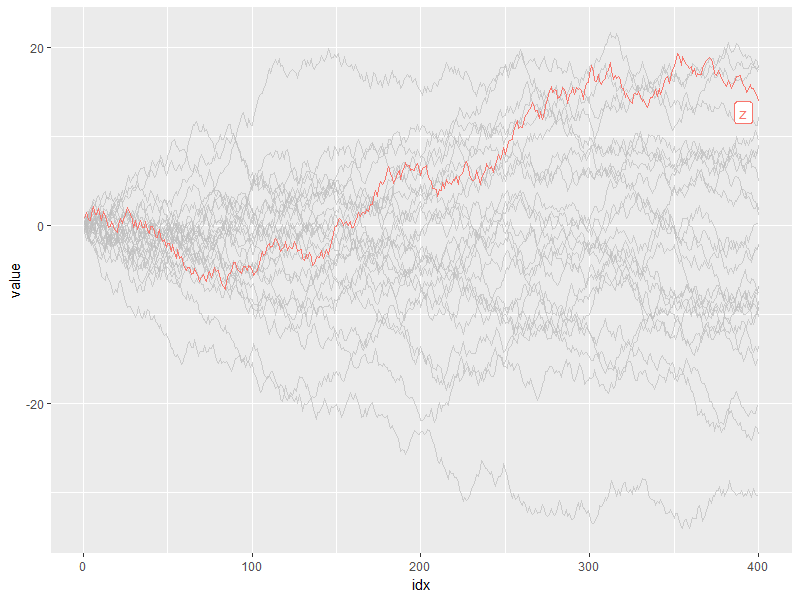## Customization

As adding gghighlight() results in a ggplot object, it is fully customizable just as we usually do with ggplot2 like custom themes.

ggplot(d) +
geom_line(aes(idx, value, colour = type)) +
gghighlight(max(value) > 19) +
theme_minimal()
#> label_key: type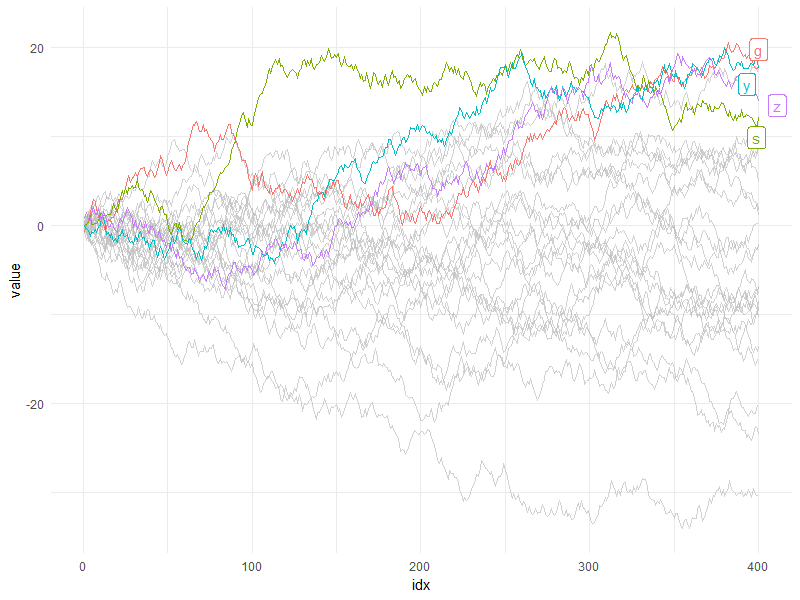The plot also can be facetted:

ggplot(d) +
geom_line(aes(idx, value, colour = type)) +
gghighlight(max(value) > 19) +
theme_minimal() +
facet_wrap(~ type)
#> label_key: type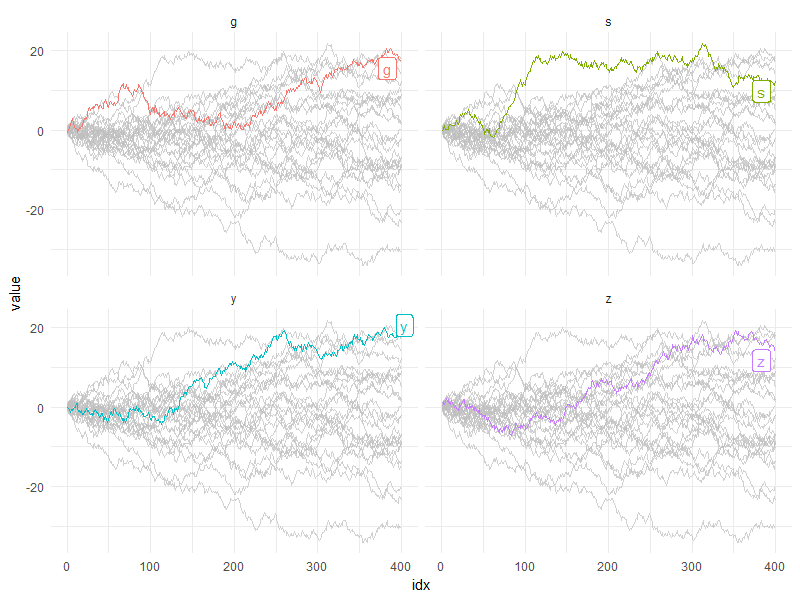## Geoms

gghighlight() can highlight almost every geoms. Here are some examples.

### Bar

gghighlight() can highlight bars.

p <- ggplot(iris, aes(Sepal.Length, fill = Species)) +
geom_histogram() +
gghighlight()
#> label_key: Species

p
#> stat_bin() using bins = 30. Pick better value with binwidth.
#> stat_bin() using bins = 30. Pick better value with binwidth.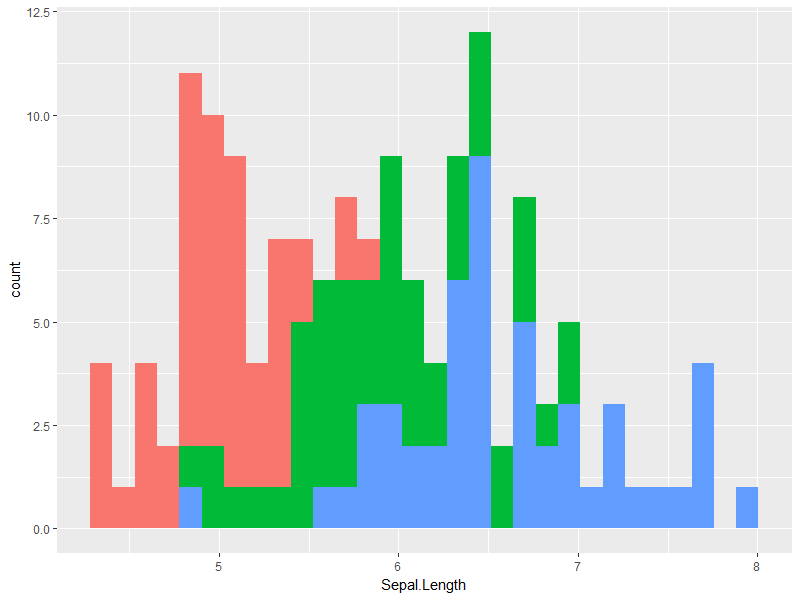You may wonder if this is really highlighted. Yes, it is. But, the unhighlighted bars are all overwritten by the highlighted bars. This seems not so useful, until you see the fecetted version:

p + facet_wrap(~ Species)
#> stat_bin() using bins = 30. Pick better value with binwidth.
#> stat_bin() using bins = 30. Pick better value with binwidth.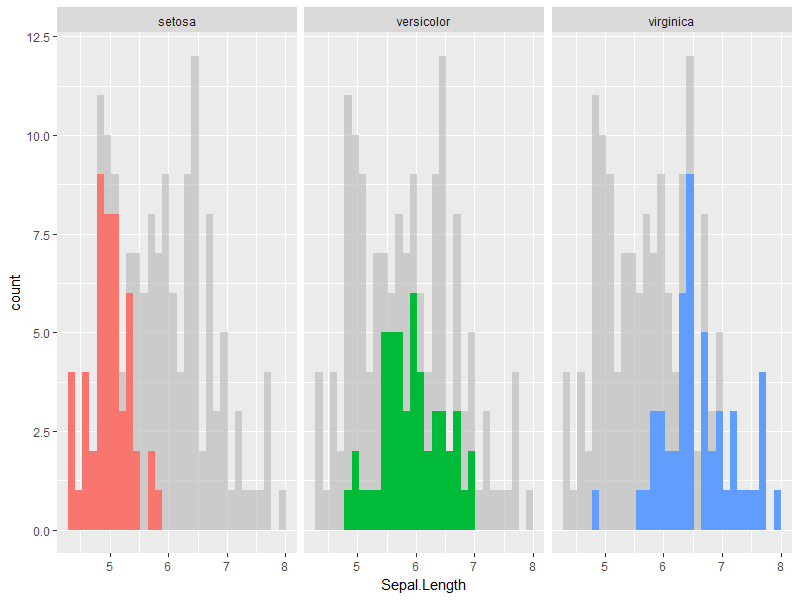### Point

As I explained in Anatomy of gghighlight, lines and points typically have different semantics (group-wise or not). But, in most cases, you don’t need to be careful about the difference with gghighlight() because it automatically picks the right way of calculation.

set.seed(10)
d2 <- dplyr::sample_n(d, 20)

ggplot(d2, aes(idx, value)) +
geom_point() +
gghighlight(value > 0, label_key = type)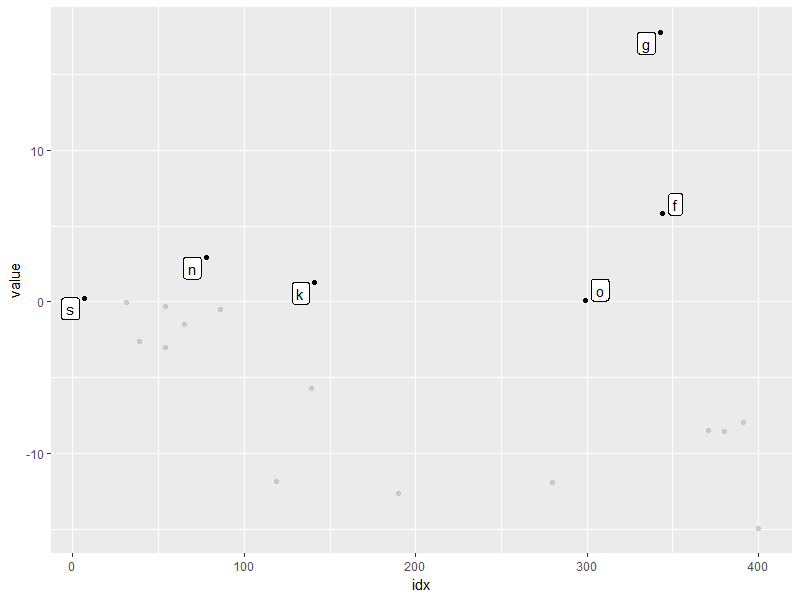More precisely, gghighlight() takes the following strategy:

1. Calculate the group IDs from mapping. a. If group exists, use it. b. Otherwise, assign the group IDs based on the combination of the values of discrete variables.
2. If the group IDs exists, evaluate the predicates in a grouped manner.
3. If the group IDs doesn’t exist or the grouped calculation fails, evaluate the predicates in an ungrouped manner.

Note that, in this case, label_key = type is needed to show labels because gghighlights() chooses a discrete variable from the mapping, but aes(idx, value) consists of only continuous variables.

### Sf

For the proof of gghighlight’s capability, here’s highlighted geom_sf():

nc <- sf::st_read(system.file("shape/nc.shp", package = "sf"), quiet = TRUE)

ggplot(nc) +
geom_sf(aes(fill = AREA)) +
gghighlight(grepl("^[A-C]", NAME)) +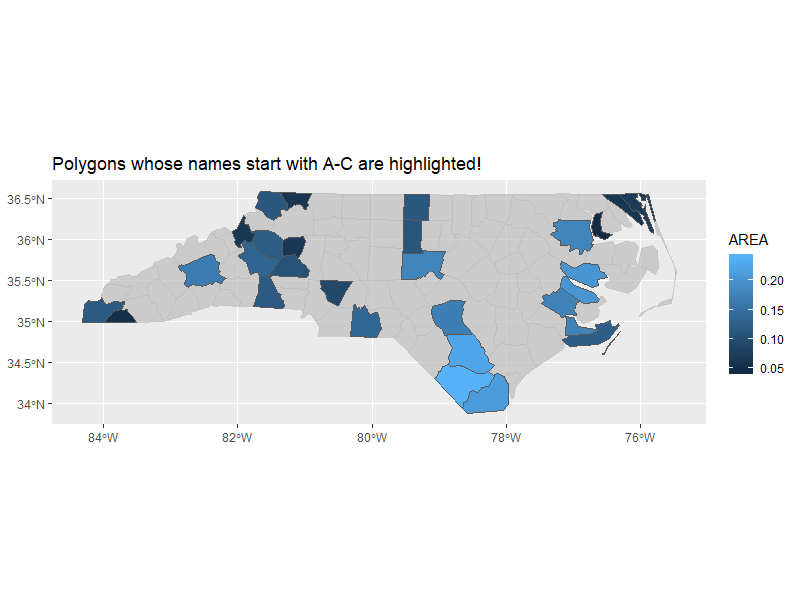### (Exceptions)

I’ve written “gghighlight() can highlight almost every geoms.” I mean, there are some exceptions that gghighlight can not handle. But, I think I’m aware of only few of these. So, please let me know if you see counter-intuitive results or errors via GitHub or Twitter or SO!

## Non-logical predicate

To construct a predicate expression like bellow, we need to determine a threshold (in this example, 20). But it is difficult to choose a nice one before we draw plots.

max(value) > 20


So, gghighlight() allows predicates that return numeric (or character) results. The values are used for sorting data and the top max_highlight of rows/groups are highlighted:

ggplot(d, aes(idx, value, colour = type)) +
geom_line() +
gghighlight(max(value), max_highlight = 5L)
#> label_key: type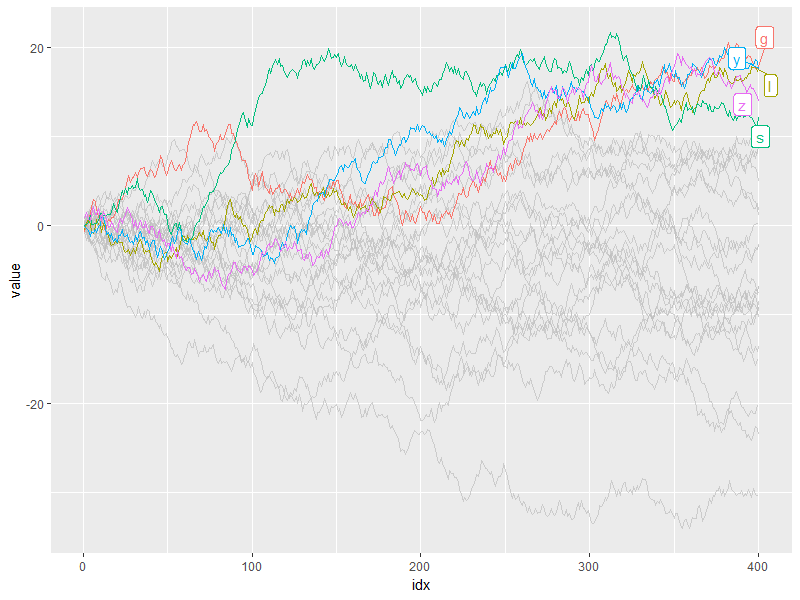## Backward-compatibility

gghighlight_point() and gghighlight_line() are here to stay for some time, but they will be deprecated in favor of gghighlight(). The design of them was due to the limitation of extendability of ggplot’s + operator, so it was not what it should be. Please consider using gghighlight() instead.

## Caveats

gghighlight is good to explore data by changing a threshold little by little. But, the internals are not so efficient, as it does almost the same calculation every time you execute gghighlight(), which may get slower when it works with larger data. Consider doing this by using vanilla dplyr to filter data.

## Alternative

FWIW, here’s a different approach of highlighting ggplot2 by atusy. While my package modifies the clones of the existing layers, ggAtusy package modifies ggproto and create a new function that creates layers. This approach seems clean and simple, whereas my code is full of tweaks and some kind of black magics…

## Summary

gghighlight package has become cool. Please try!

Bug reports or feature requests are welcome! -> https://github.com/yutannihilation/gghighlight/issues